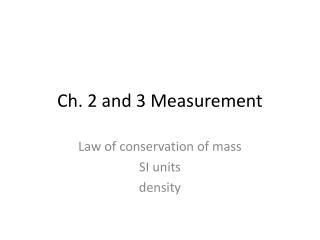DownloadDownload PresentationCh. 2 and 3 Measurement

# Ch. 2 and 3 Measurement

Download Presentation## Ch. 2 and 3 Measurement

- - - - - - - - - - - - - - - - - - - - - - - - - - - E N D - - - - - - - - - - - - - - - - - - - - - - - - - - -
##### Presentation Transcript

1. Ch. 2 and 3 Measurement Law of conservation of mass SI units density

2. Chemical Symbols • When writing the chemical symbol for an element, the first letter is always capitalized, and the second letter (if there is one) is always lower case.

3. Chemical Equations • Reactants  Products • Reactants are what goes into the reaction •  means yields or produces • Products are what result from a chemical reaction

4. Law of Conservation of Mass • Mass of reactants equals the mass of the products, because mass cannot be created or destroyed by chemical reactions (only nuclear reactions) • Ex. If 25 grams of aluminum react with 5 grams of oxygen, how many grams of aluminum oxide are produced?

5. SI Units Symbol Base Unit Abbrev. Quantity Length l meter m Mass m kilogram kg Volume v cm3 cubic centimeter Temp T kelvin K

6. mega- kilo- k M 106 103 deci- d 10-1 centi- c 10-2 milli- m 10-3 micro-  10-6 nano- n 10-9 pico- p 10-12 Selected Prefixes for Metric Units Prefix Symbol Factor 100 - - - BASE UNIT

7. Density The ratio between the object’s mass and its volume.

8. Density • Density = • Density is a characteristic (physical) property that depends only on the composition of a substance, not on the size of the sample. • The composition of a mixture can vary therefore the density can vary. Mass Volume M V D =

9. Density Mass (g) Volume (cm3)

10. A copper penny has a mass of 3.1g and a volume of 0.35 cm3. What is the density of copper? Density = mass volume Density = 3.1g 0.35 cm3 Density practice problem = 8.8571 g/cm3 = 8.9 g/cm3